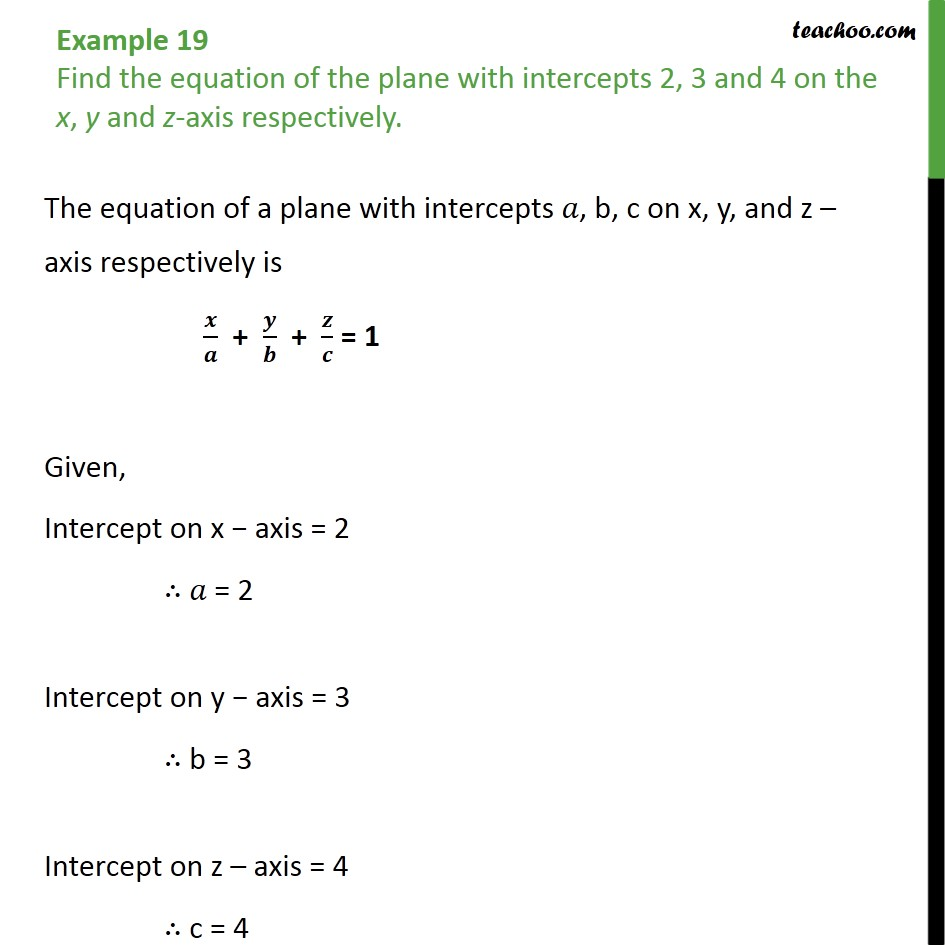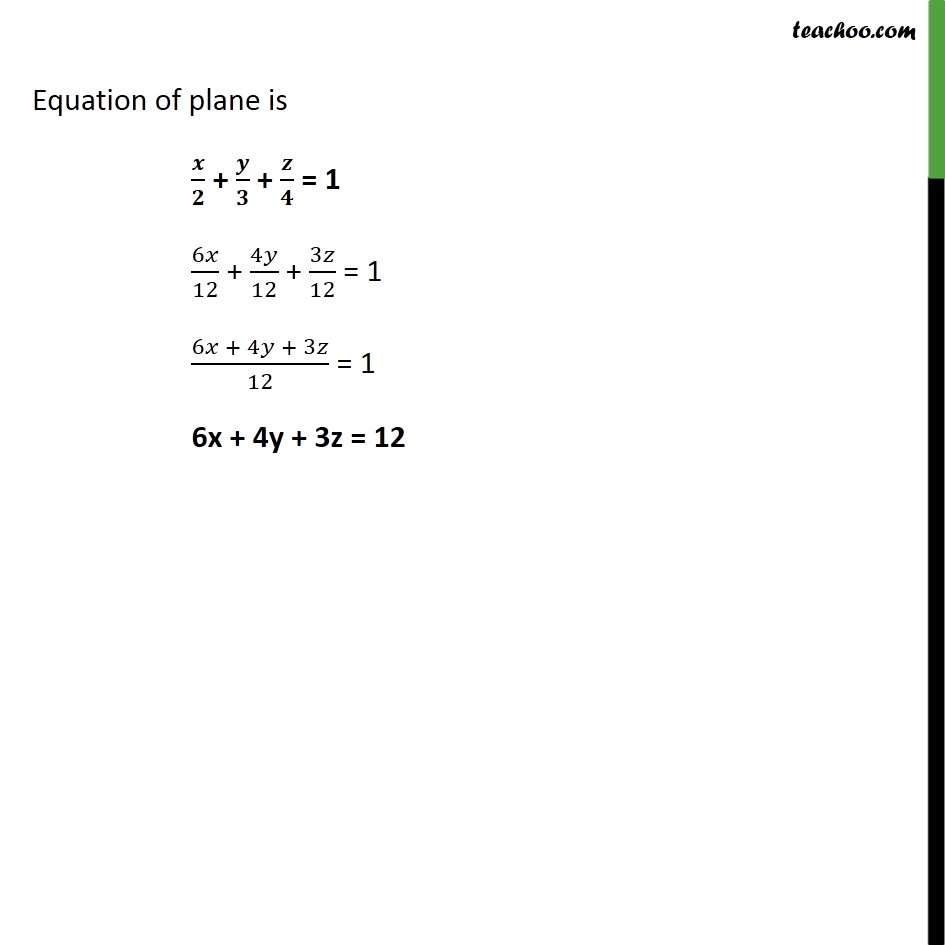1. Chapter 11 Class 12 Three Dimensional Geometry
2. Concept wise
3. Equation of plane - Intercept Form

Transcript

Example 19 Find the equation of the plane with intercepts 2, 3 and 4 on the x, y and z-axis respectively. The equation of a plane with intercepts 𝑎, b, c on x, y, and z – axis respectively is 𝒙﷮𝒂﷯ + 𝒚﷮𝒃﷯ + 𝒛﷮𝒄﷯ = 1 Given, Intercept on x − axis = 2 ∴ 𝑎 = 2 Intercept on y − axis = 3 ∴ b = 3 Intercept on z – axis = 4 ∴ c = 4 Equation of plane is 𝒙﷮𝟐﷯ + 𝒚﷮𝟑﷯ + 𝒛﷮𝟒﷯ = 1 6𝑥﷮12﷯ + 4𝑦﷮12﷯ + 3𝑧﷮12﷯ = 1 6𝑥 + 4𝑦 + 3𝑧﷮12﷯ = 1 6x + 4y + 3z = 12

Equation of plane - Intercept Form

About the AuthorDavneet Singh
Davneet Singh is a graduate from Indian Institute of Technology, Kanpur. He has been teaching from the past 9 years. He provides courses for Maths and Science at Teachoo.